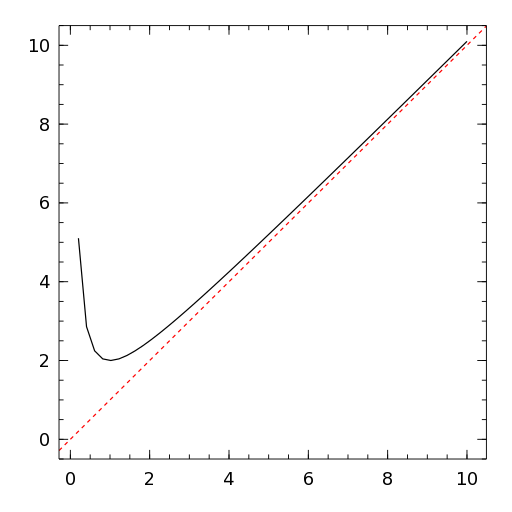# Slope¶

Slope(slope, intercept, args...; kvs...)

PlotComponent to draw a straight line with slope slope. The vertical offset is given by intercept which must be a tuple of length 2 containing the coordinates of a point on the line. args and kvs can be used to set additional style attributes.

## Example¶

x = linspace(0, 10)
p = plot(x, x + 1 ./ x, yrange=[-0.5, 10.5])
s = Slope(1, (5, 5), color="red", linekind="dashed")